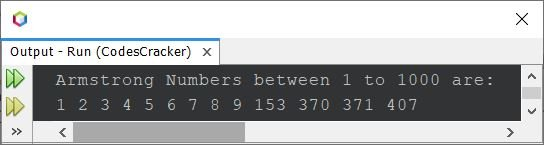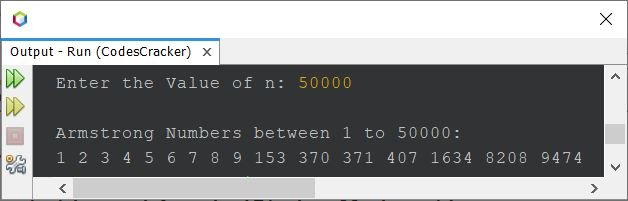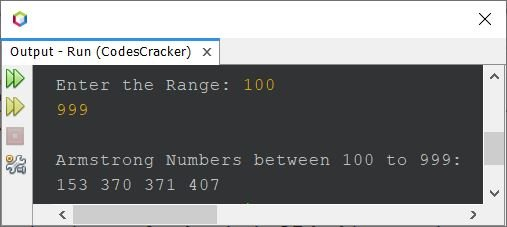# Java Program to Find and Print Armstrong Numbers

• Print Armstrong Numbers between 1 to 1000
• Print Armstrong Numbers between 1 to n
• Print Armstrong Numbers withing a given range (m to n)

A number that is equal to the sum of its own digits, where each digit raised to the power of number of digit is called as an Armstrong number. For example, 407 is an Armstrong number, because:

```407 = 43 + 03 + 73
= 64 + 0 + 343
= 407```

Some other Armstrong numbers are 1, 2, 3, 4, 5, 6, 7, 8, 9, 153, 370, 371, 1634 etc.

## Find Armstrong Numbers between 1 to 1000 in Java

The question is, write a Java program to find and print all Armstrong numbers between 1 to 1000. The program given below is its answer:

```import java.util.Scanner;

public class CodesCracker
{
public static void main(String[] args)
{
int val, i, totalDigit, r, temp, rem, pow, k;

System.out.println("Armstrong Numbers between 1 to 1000 are:");
for(i=1; i<=1000; i++)
{
totalDigit=0;
r=0;
val = i;
temp = val;
while(temp>0)
{
totalDigit++;
temp = temp/10;
}
temp = val;
while(temp>0)
{
rem = temp%10;
pow = 1;
k = 0;
while(k<totalDigit)
{
pow = pow*rem;
k++;
}
r = r+pow;
temp = temp/10;
}
if(r==val)
System.out.print(r+ " ");
}
}
}```

The snapshot given below shows the sample output produced by above Java program, that prints all Armstrong numbers between 1 to 1000:The above program can also be created in this way. This program uses for loop, in place of while loop. Also, all the extra statements/codes are removed:

```import java.util.Scanner;
import java.lang.Math;

public class CodesCracker
{
public static void main(String[] args)
{
int i, totalDigit, r, temp, rem;

System.out.println("Armstrong Numbers between 1 to 1000 are:");
for(i=1; i<=1000; i++)
{
totalDigit=0;
r=0;
for(temp=i; temp>0; temp /= 10)
totalDigit++;
for(temp=i; temp>0; temp /= 10)
r = r + (int) Math.pow((temp%10), totalDigit);
if(r==i)
System.out.print(r+ " ");
}
}
}```

This program produces same output as of previous program.

## Print Armstrong Number between 1 to n in Java

The question is, write a program in Java to find and print Armstrong numbers between 1 to n. The value of n must be received by user at run-time. The program given below, is its answer:

```import java.util.Scanner;
import java.lang.Math;

public class CodesCracker
{
public static void main(String[] args)
{
int n, i, totalDigit, r, temp, rem;

Scanner scan = new Scanner(System.in);

System.out.print("Enter the Value of n: ");
n = scan.nextInt();

System.out.println("\nArmstrong Numbers between 1 to " +n+ ":");
for(i=1; i<=n; i++)
{
totalDigit=0;
r=0;
for(temp=i; temp>0; temp /= 10)
totalDigit++;
for(temp=i; temp>0; temp /= 10)
r = r + (int) Math.pow((temp%10), totalDigit);
if(r==i)
System.out.print(r+ " ");
}
}
}```

The sample run of above program with user input 50000 as value of n to print all Armstrong numbers from 1 to 50000, is shown in the snapshot given below:## Find Armstrong Numbers within a Range in Java

This is the last program of this article, created to print Armstrong numbers within a particular range.

```import java.util.Scanner;
import java.lang.Math;

public class CodesCracker
{
public static void main(String[] args)
{
int start, end, i, totalDigit, r, temp, rem;

Scanner scan = new Scanner(System.in);

System.out.print("Enter the Range: ");
start = scan.nextInt();
end = scan.nextInt();

System.out.println("\nArmstrong Numbers between " +start+" to " +end+ ":");
for(i=start; i<=end; i++)
{
totalDigit=0;
r=0;
for(temp=i; temp>0; temp /= 10)
totalDigit++;
for(temp=i; temp>0; temp /= 10)
r = r + (int) Math.pow((temp%10), totalDigit);
if(r==i)
System.out.print(r+ " ");
}
}
}```

Here is its sample run with user input 100 and 999 as range to find and print all Armstrong numbers between 100 to 999:#### Same Program in Other Languages

Java Online Test

« Previous Program Next Program »